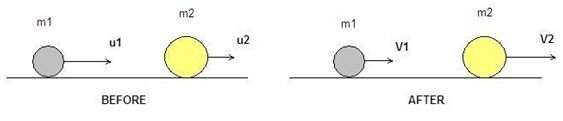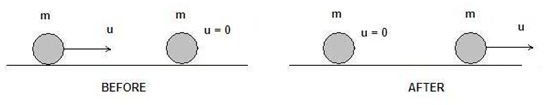# Explanation of How to Solve Elastic Collision Theory Problems

## Types of Collisions – What is an Elastic Collision?

In physics, a collision is defined as the phenomenon in which a momentum and energy transfer takes place between two or more objects. There are two main types of collisions as far as energy transfer is concerned; the elastic and inelastic collision. During an elastic collision, the momentum and the kinetic energy of the system are conserved. Collisions between hard steel balls, atomic particles and pool balls are considered to be elastic. During an inelastic collision though, only the momentum of the system is conserved. A part of the original kinetic energy is converted to some other form of energy or heat after the collision takes place.

The article describes the steps on how to solve elastic collision problems. In case you are interested to learn more on how to solve inelastic and plastic collision problems, check out the article, How to Solve Inelastic and Plastic Collision Problems.

## Mathematical Background – Energy and Momentum Conservation Principles

The use of conservation laws in elastic collision theory is a useful tool for solving elastic collision problems.

Lets assume that we have a system of two ideal particles with masses m1 and m2 moving in two dimensions. No external forces are acting on the system (closed) and the two masses have initial velocities u1 and u2 respectively. After the collision they will have velocities V1 and V2. The use of bold letters denotes that the velocities are vectors.

According to the conservation of momentum principle, the momentum pBEFORE before the collision is equal to the momentum pAFTER after the collision:

pBEFORE = pAFTER =>

p 1,BEFORE + p 2,BEFORE = p 1,AFTER + p 2,AFTER =>

m1u1 + m2u2 = m1V1 + m2V2 (1)

For more information on the conservation of momentum principle, check out the article, Understanding the Conservation of the Momentum Principle.

According to the conservation of kinetic energy, the kinetic energy KBEFORE before the collision is equal to the kinetic energy KAFTER after the collision:

KBEFORE = KAFTER =>

K 1,BEFORE + K 2,BEFORE = K 1,AFTER + K 2,AFTER =>

(1/2)m1u12 +(1/2)m2u22 = (1/2)m1V12 +(1/2)m2V22 (2)

## Steps to Solve an Elastic Collision Problem in Two Dimensions

Below there are some simple directions that explain how to solve an elastic collision problem in the two dimensions. Lets assume that we need to calculate the velocities V1 and V2 of the two masses, after the elastic collision has taken place:

• The first step is to design the vectors of velocity for each of the bodies before and after the collision.
• Choose the positive direction, usually toward the right. This helps us to define the sign of velocities ( + or – ) in the equations.
• Write the conservation of momentum and kinetic energy principles or the expressions (1) and (2) from above.
• Solve the system of equations (1) and (2). The result will be an expression that combines the velocities before and after the collision:

u1u2 = – (V1V2) (3)

We do this, because it is easier to work with linear equations rather than quadratic.

• Then solve the system of (1) and (3):

V1 = [2m2u2+(m1-m2)u1]/(m1+m2)

V2 =[2m1u1+(m2-m1)u2]/(m1+m2)

We have finally come to calculate the final velocities of the masses. In a similar way we can calculate the initial velocities instead, as long as all other parameters are known.

Image: The diagram shows an elastic collision between two objects. Click to enlarge.Special case: When the two objects have equal masses m1 = m2 = m the solution of the (1) and (3) system results in:

V1 = u2 and V2 = u1

We observe that the two objects exchange velocities.

Image: The diagram shows an elastic collision between two objects of the same mass m. The two objects exchange velocities. Click to enlarge.Special case: When one of the objects is not moving (u2 = 0), the solution of the (1) and (3) system results in the following:

V1 = [(m1-m2)u1]/(m1+m2)

V2 = 2m1u1/(m1+m2)

## Neutron Deceleration Inside the Nuclear Reactors

The case in which the masses of the two particles are equal m1 = m2 = m and one of them is not moving (u2 = 0), is applicable during the neutron deceleration that takes place within a nuclear reactor. Water is used to decelerate the high energy neutrons. The neutron mass mn is almost equal to the proton mass mp. So, when a neutron hits a resting hydrogen nucleus (or a proton), it will transfer almost all of its kinetic energy to the hydrogen nucleus and the resulting low energy neutron will not be able to initiate further nuclear reactions.

## Sources

• physics.ucsc.edu
• hyperphysics.phy-astr.gsu.edu
• plasmaphysics.org.uk
• Screenshots by author